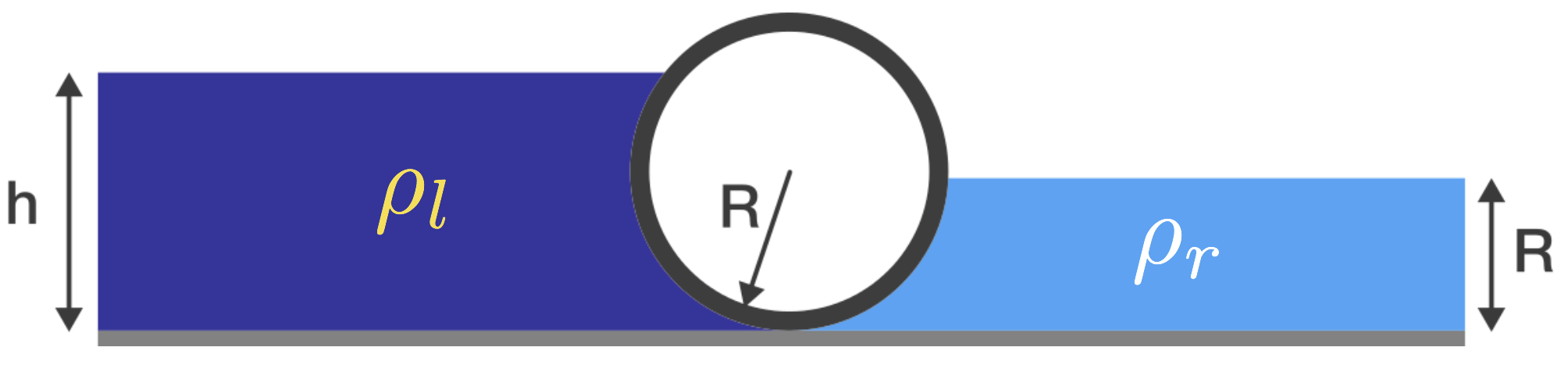# The Ball in Liquid columns CoulumnsA heavy cylinder of radius $R$ lies in equilibrium on a smooth surface, separating two liquids of densities $\rho_l$ on the left and $\rho_r$ on the right, with $\rho_r > \rho_l.$

If the height of the liquid on the right is $R,$ what is the height $h$ of the liquid on the left in terms of $R?$

Neglect the surface tensions of the liquids.

×